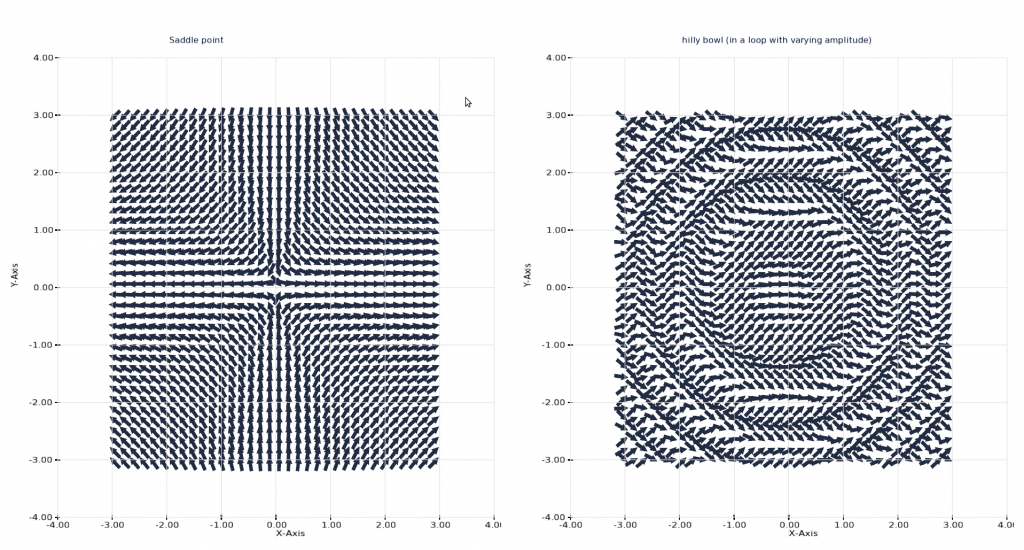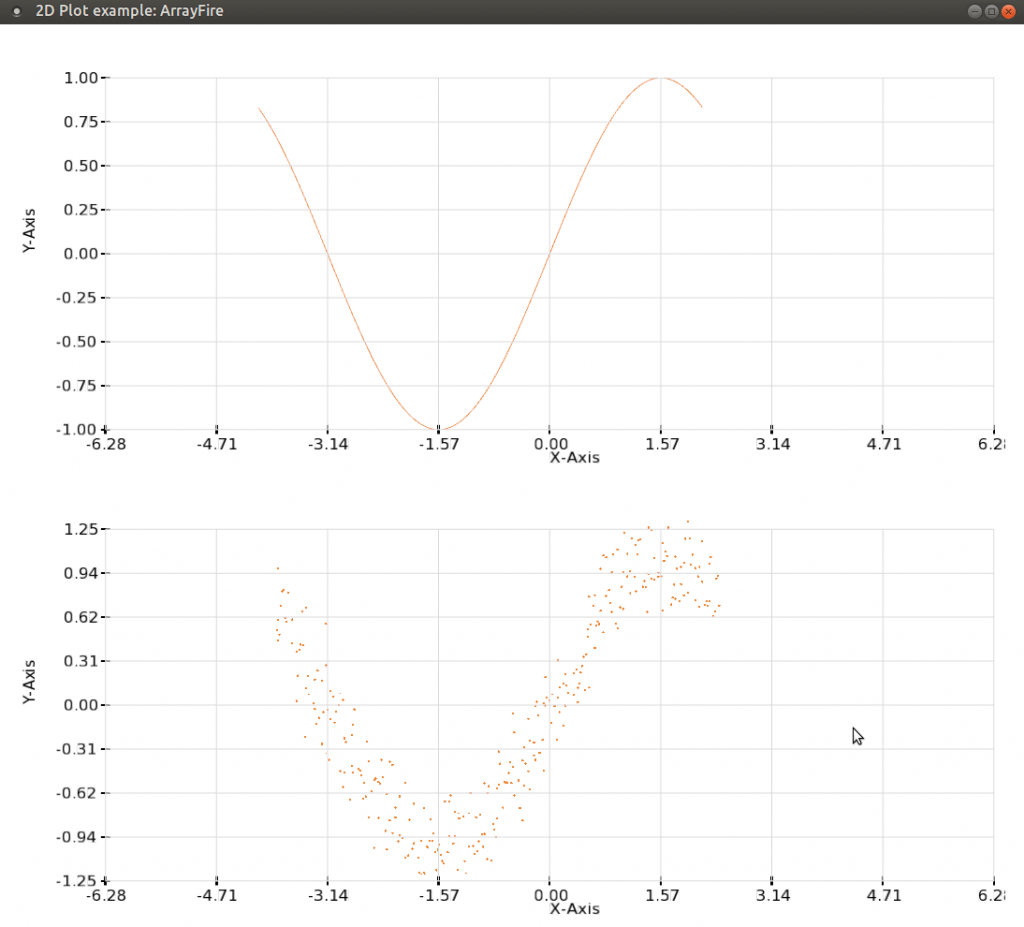# Graphics Updates in ArrayFire v3.4

This post outlines the new graphics features available in ArrayFire v3.4:  Vector Fields, Overlays

### Vector Fields

We have added visualization support to render ArrayFire array objects as vector fields. An example of how to visualize vector fields is included in ArrayFire v3.4. A screenshot of this example’s output in multi-view mode is shown below, showcasing both static and dynamic vector field rendering.### Overlays

Previously, each graph (such as plot, hist, scatter, etc) was rendered in its own window (or view). Overlaying graphs was not supported. ArrayFire v3.4 now support graph overlays.

Each draw call in ArrayFire is either rendered to a whole window (single view) or to a view, which is a portion of the screen obtained in multiview mode.

The following image is an example of a graph overlay:The following graphs support overlay if they are of same type of object (2d/3d):

### Animations

ArrayFire v3.4 includes the following examples of producing fun animations based on simple equations: conway, conway_pretty, gravity, and julia fractal.

### Behavioral Changes

In previous versions of ArrayFire, axes limits were computed automatically to fit the data. In ArrayFire v3.4, users are required to set axes limits, as shown below:

``````
af::Window myWindow(800, 800, "2D Plot example: ArrayFire");

array Y;
int sign = 1;
array X = seq(-af::Pi, af::Pi, PRECISION);
array noise = randn(X.dims(0))/5.f;

myWindow.grid(2, 1);
// Set the axes limits to view (0,0), prior to v3.4 this was not needed
myWindow(0, 0).setAxesLimits(-2 * af::Pi, 2 * af::Pi, -1, 1, true);
// Set the axes limits to view (0,0), prior to v3.4 this was not needed
myWindow(1, 0).setAxesLimits(-2 * af::Pi, 2 * af::Pi, -1.25, 1.25, true);

for (double val=0; !myWindow.close(); )
{
Y = sin(X);

myWindow(0,0).plot(X, Y);
myWindow(1,0).scatter(X, Y + noise, AF_MARKER_POINT);

myWindow.show();

X = X + PRECISION * float(sign);
val += PRECISION * float(sign);

if (val >af::Pi) {
sign = -1;
} else if (val < -af::Pi) {
sign = 1;
}
}
``````

The code above produces the output below. You can find the complete example here.Miscellaneous Change

Removed GLEW and replaced with glbinding.